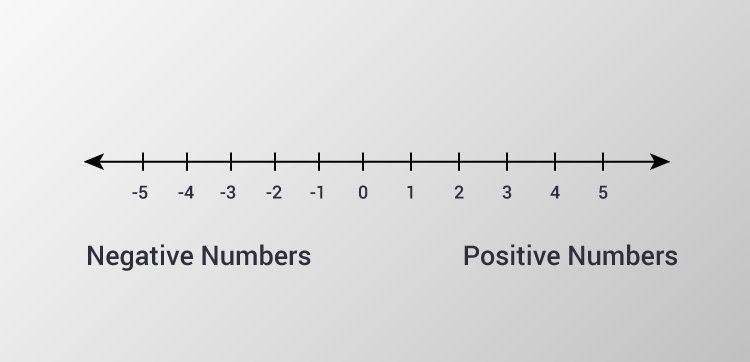# C Program to Check Whether a Number is Positive or Negative

In this example, you will learn to check whether a number (entered by the user) is negative or positive.To understand this example, you should have the knowledge of following C programming topics:

This program takes a number from the user and checks whether that number is either positive or negative or zero.

## Example #1: Check if a Number is Positive or Negative Using if...else

``````#include <stdio.h>
int main()
{
double number;

printf("Enter a number: ");
scanf("%lf", &number);

if (number <= 0.0)
{
if (number == 0.0)
printf("You entered 0.");
else
printf("You entered a negative number.");
}
else
printf("You entered a positive number.");
return 0;
}```
```

You can also solve this problem using nested if else statement.

## Example #2: Check if a Number is Positive or Negative Using Nested if...else

``````#include <stdio.h>
int main()
{
double number;

printf("Enter a number: ");
scanf("%lf", &number);

// true if number is less than 0
if (number < 0.0)
printf("You entered a negative number.");

// true if number is greater than 0
else if ( number > 0.0)
printf("You entered a positive number.");

// if both test expression is evaluated to false
else
printf("You entered 0.");
return 0;
}
``````

Output 1

```Enter a number: 12.3
You entered a positive number.
```

Output 2

```Enter a number: 0
You entered 0.
```